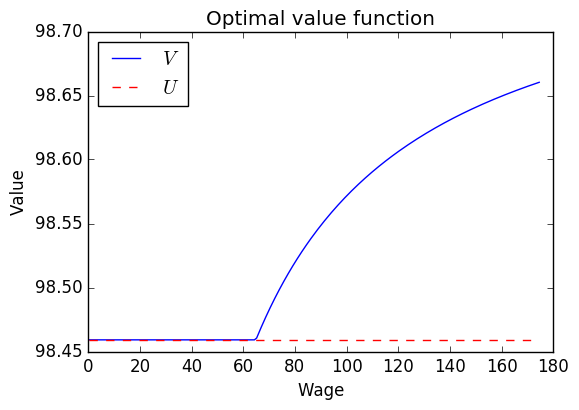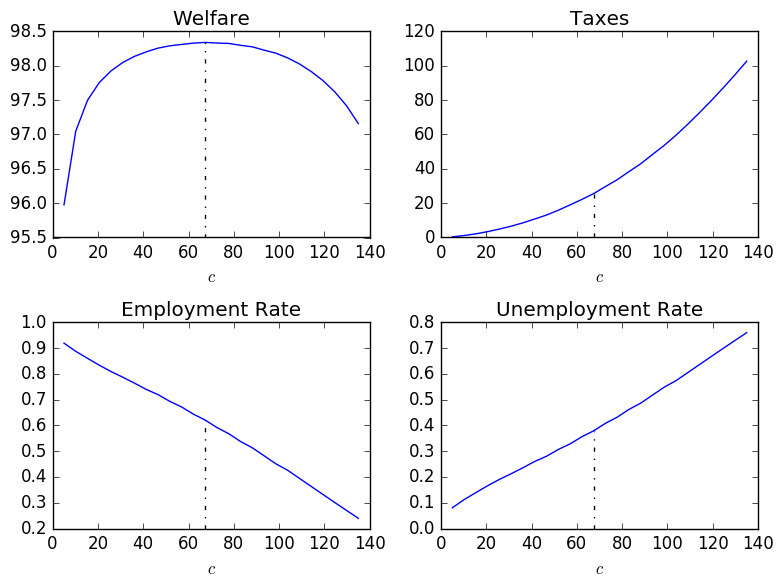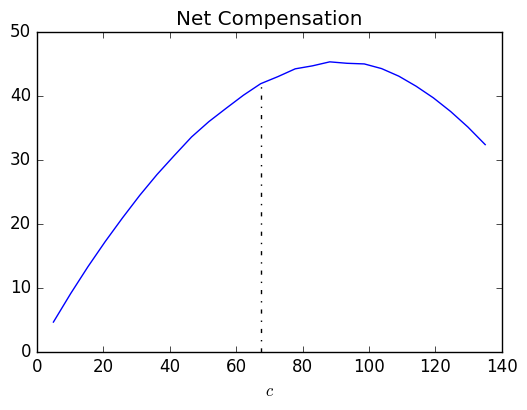# DiscreteDP Example: Job Search¶

Daisuke Oyama

Faculty of Economics, University of Tokyo

We study an optimal stopping problem, in the context of job search as discussed in http://quant-econ.net/py/lake_model.html.

In :
using QuantEcon
import QuantEcon: solve
using Distributions
using PyPlot
using Roots


## Optimal solution¶

We skip the description of the model, just writing down the Bellman equation: \begin{aligned} U &= u(c) + \beta \left[(1 - \gamma) U + \gamma E[V_s]\right], \\ V_s &= \max\left\{U, u(w_s) + \beta \left[(1 - \alpha) V_s + \alpha U\right] \right\}. \end{aligned} For this class of problem, we can characterize the solution analytically.

The optimal policy $\sigma^*$ is monotone; it is characterized by a threshold $s^*$, for which $\sigma^*(s) = 1$ if and only if $s \geq s^*$, where actions $0$ and $1$ represent "reject" and "accept", respectively. The threshold is defined as follows: Let \begin{aligned} g(s) &= u(w_s) - u(c), \\ h(s) &= \frac{\beta \gamma}{1 - \beta (1 - \alpha)} \sum_{s' \geq s} p_s u(w_s). \end{aligned} It is easy to see that $g$ is increasing and $h$ is decreasing. Then the threshold $s^*$ is such that $s \geq s^*$ if and only if $g(s) > h(s)$.

Given $s^*$, the optimal values can be computed as follows: \begin{aligned} U &= \frac{\{1 - (1 - \alpha) \beta\} u(c) + \beta \gamma \sum_{s \geq s^*} p_s u(w_s)} {(1 - \beta) \left[\{1 - (1 - \alpha) \beta\} + \beta \gamma \sum_{s \geq s^*} p_s\right]}, \\ V_s &= \begin{cases} U & \text{if s < s^*} \\ \dfrac{u(w_s) + \alpha \beta U}{1 - (1 - \alpha) \beta} & \text{if s \geq s^*}. \end{cases} \end{aligned}

The optimal policy defines a Markov chain over $\{\text{unemployed}, \text{employed}\}$. Its stationary distribution is $\pi = \left(\frac{\alpha}{\alpha + \lambda}, \frac{\lambda}{\alpha + \lambda}\right)$, where $\lambda = \gamma \sum_{s \geq s^*} p(w_s)$; note that the flow from unemployed to employed is $\lambda$, while the flow from employed to unemployed is $\alpha$.

The expected value at the stationary distribution is $$\pi_0 U + \pi_1 \frac{\sum_{s \geq s^*} p_s V_s}{\sum_{s \geq s^*} p_s}.$$

The above analytical solution aside, we solve the job search problem using the DiscreteDP type:

In :
type JobSearchModel
# Parameters
w::Vector{Float64}
w_pdf::Vector{Float64}
beta::Float64
alpha::Float64
gamma::Float64
rho::Float64
# Internal variables
u::Function
ddp::DiscreteDP
num_states::Integer
num_actions::Integer
rej::Integer
acc::Integer

function JobSearchModel(w::Vector, w_pdf::Vector, beta::Float64;
alpha::Float64=0., gamma::Float64=1., rho::Float64=0.)
# Utility function
function u(y::Vector{Float64})
small_number = -9999999
nonpositive = (y .<= 0)
if rho == 1
util = log(y)
else
util = (y.^(1 - rho) - 1)/(1 - rho)
end
util[nonpositive] = small_number
return util
end
u_w = u(w)

num_states = length(w) + 1
num_actions = 2
rej, acc = 1, 2

# Reward array
R0 = zeros(num_states, num_actions)
R0[1:end-1, acc] = u_w

# Transition probability array
Q = zeros(num_states, num_actions, num_states)
# Reject
for s in 1:num_states
Q[s, rej, 1:end-1] = w_pdf * gamma
Q[s, rej, end] = 1 - gamma
end
# Accept
for s in 1:num_states-1
Q[s, acc, s] = 1 - alpha
Q[s, acc, end] = alpha
end
Q[end, acc, 1:end-1] = w_pdf

ddp = DiscreteDP(R0, Q, beta)

js = new(w, w_pdf, beta, alpha, gamma, rho, u, ddp,
num_states, num_actions, rej, acc)
return js
end
end;

In :
function solve(js::JobSearchModel, c::Float64)
n, m = js.num_states, js.num_actions
rej = js.rej
js.ddp.R[:, rej] = js.u([c])
js.ddp.R[end, :] = js.u([c])

res = solve(js.ddp, PFI)
V::Vector{Float64} = res.v[1:end-1]  # Values of jobs
U::Float64 = res.v[end]  # Value of unemployed
C::Vector{Int64} = res.sigma[1:end-1] - 1  # Optimal policy

lamb::Float64 = dot(js.w_pdf, C) * js.gamma
pi::Vector{Float64} = [js.alpha, lamb]
pi /= sum(pi)  # Stationary distribution

return V, U, C, pi
end;


The following paramter values are from lakemodel_example.py.

In :
w = linspace(0, 175, 201)  # wage grid

# compute probability of each wage level
logw_dist = Normal(log(20), 1)
logw_dist_cdf = cdf(logw_dist, log(w))
logw_dist_pdf = logw_dist_cdf[2:end] - logw_dist_cdf[1:end-1]
logw_dist_pdf /= sum(logw_dist_pdf)
w = Array((w[2:end] + w[1:end-1])/2);

In :
gamma = 1.
alpha = 0.013  # Monthly
alpha_q = (1-(1-alpha)^3)  # Quarterly
beta = 0.99
rho = 2.  # risk-aversion;

In :
js = JobSearchModel(w, logw_dist_pdf, beta, alpha=alpha_q, gamma=gamma, rho=rho);


Take a look at the optimal solution for $c = 40$ for example:

In :
c = 40.
V, U, C, pi = solve(js, c);

In :
s_star = length(w) - sum(C) + 1
println("Optimal policy: Accept if and only if w >= $(w[s_star])")  Optimal policy: Accept if and only if w >= 65.1875  In : fig, ax = subplots(figsize=(6, 4)) ax[:plot](w, V, label=L"$V$") ax[:plot]((w, w[end]), (U, U), "r--", label=L"$U$") ax[:set_title]("Optimal value function") ax[:set_xlabel]("Wage") ax[:set_ylabel]("Value") legend(loc=2) show()## Optimal unemployment insurance policy¶ We compute the optimal level of unemployment insurance as in the lecture, mimicking lakemodel_example.py. In : type UnemploymentInsurancePolicy w::Vector{Float64} w_pdf::Vector{Float64} beta::Float64 alpha::Float64 gamma::Float64 rho::Float64 function UnemploymentInsurancePolicy(w::Vector, w_pdf::Vector, beta::Float64; alpha::Float64=0., gamma::Float64=1., rho::Float64=0.) uip = new(w, w_pdf, beta, alpha_q, gamma, rho) return uip end end;  In : function solve_job_search_model(uip::UnemploymentInsurancePolicy, c::Float64, T::Float64) js = JobSearchModel(uip.w-T, uip.w_pdf, uip.beta, alpha=uip.alpha, gamma=uip.gamma, rho=uip.rho) V, U, C, pi = solve(js, c-T) return V, U, C, pi end;  In : function budget_balance(uip::UnemploymentInsurancePolicy, c::Float64, T::Float64) V, U, C, pi = solve_job_search_model(uip, c, T) return T - pi*c end;  In : function implement(uip::UnemploymentInsurancePolicy, c::Float64) # Budget balancing tax given c T = fzero(T -> budget_balance(uip, c, T), 0., c, xtolrel=1e-3) V, U, C, pi = solve_job_search_model(uip, c, T) EV = dot(C .* V, uip.w_pdf) / (dot(C, uip.w_pdf)) W = pi * U + pi * EV return T, W, pi end;  In : uip = UnemploymentInsurancePolicy(w, logw_dist_pdf, beta, alpha=alpha_q, gamma=gamma, rho=rho);  In : grid_size = 26 cvec = linspace(5, 135, grid_size) Ts, Ws = Array(Float64, grid_size), Array(Float64, grid_size) pis = Array(Float64, 2, grid_size) for (i, c) in enumerate(cvec) T, W, pi = implement(uip, c) Ts[i], Ws[i], pis[:, i] = T, W, pi end i_max = indmax(Ws) println("Optimal unemployment benefit:$(cvec[i_max])")

Optimal unemployment benefit: 67.4

In :
function plot(ax, y_vec, title)
ax[:plot](cvec, y_vec)
ax[:set_xlabel](L"$c$")
ax[:vlines](cvec[i_max], ax[:get_ylim](), y_vec[i_max], "k", "-.")
ax[:set_title](title)
end

fig, axes = subplots(2, 2)
plot(axes[1, 1], Ws, "Welfare")
plot(axes[1, 2], Ts, "Taxes")
plot(axes[2, 1], vec(pis[2, :]), "Employment Rate")
plot(axes[2, 2], vec(pis[1, :]), "Unemployment Rate")

tight_layout()
show()In :
fig, ax = subplots(figsize=(6, 4))
plot(ax, cvec-Ts, "Net Compensation")
show()In [ ]: